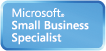Welcome Guest, you are in: Login

# MUST Creative Engineering Laboratory

### Technical Doc

Search the wiki
»

www.must.or.kr# Fourier Transform Properties

Modified on 2010/11/11 16:08 Categorized as Digital Image Processing

# Fourier Transform Properties - Separability# Fourier Transform Properties - Convolution# Fourier Transform Properties - TranslationTranslation property results in a phase shift of the spectrum. (a) Original image. (b) The magnitude of the Fourier spectrum from (a) represented as an image. (c) The phase of the Fourier spectrum from (a) represented by an image. (d) and (g) are original image shifted by 128 rows and 128 columns, and 64 rows and 64 columns, respectively. (e), (h) The magnitude spectrum. (f), (i) Phase spectrum. These images illustrate that when an image is translated, the phase changes, even though magnitude remains the same.

# Fourier Transform Properties - RotationTranslation property results in corresponding rotations with image and spectrum. (a) Original image. (b)  Fourier spectrum image of original image. (c) Original image rotated by 90 degrees. (d) Fourier spectrum image of rotated

# Fourier Transform Properties – PeriodicityPeriodicity and implied symmetry for the Fourier transform. (a) Implied symmetry with origin in upper-left corner. Each NxN block represented all the transform coefficients, and is repeated infinitely in all directions. (b) Increasing frequency in direction of arrows. (c) Periodic spectrum, with quadrants labeled A, B, C, and D. (d) Spectrum shifted to center. Frequency increases in all directions as we move away from theDirecting mapping of Fourier magnitude data. (a) Original image. (b) The Fourier magnitude directly remapped to 0-255 without any enhancement. (c)-(e) Contrast enhanced versions of (b).# Discrete Fourier Transform Program Coding

1. input image : own created image with proper size

2. program code must be your own

3. Discuss result

□ image -> DFT -> log scale transform -> Display

left : original input image middle : normal display of FFT transform right image : log display of FFT transform￭ created image : 512×512 image￭ created image : 1024×1024 image

## Code

```	for (int y = 0; y < height; y++)
{
for (int x = 0; x < width; x++)
{
minMagnitude = Math.Min(minMagnitude, data[y,x].Magnitude);
maxMagnitude = Math.Max(maxMagnitude, data[y,x].Magnitude);
minLog = Math.Min(minLog, Math.Log(data[y, x].Magnitude));
maxLog = Math.Max(maxLog, Math.Log(data[y, x].Magnitude));
}
}

// do the job
unsafe
{
byte* dst = (byte*)dstData.Scan0.ToPointer();

for (int y = 0; y < height; y++)
{
for (int x = 0; x < width; x++, dst++)
{
if (logTransform)
*dst = (byte)System.Math.Max(0, System.Math.Min(255, (Math.Log(data[y, x].Magnitude) - minLog) * 255 / (maxLog - minLog)));
else
*dst = (byte)System.Math.Max(0, System.Math.Min(255, data[y, x].Magnitude * scale * 255));
}
dst += offset;
}
}
```